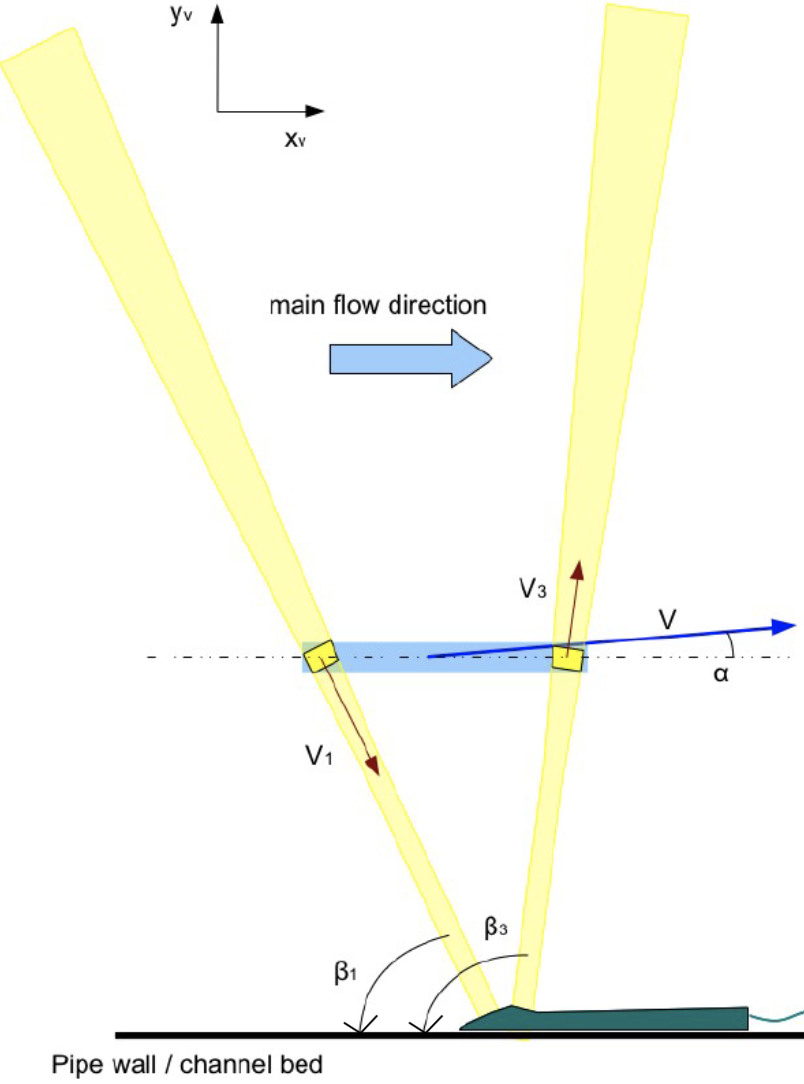### 2-3C monostatic velocity measurement

The 2C monostatic technique consists in measuring the projection of the velocity vectors over two different beam axes and in combining pairs of measuring cells in order to compute the velocity components of the profile in an orthonormal coordinate system $$(x, y)$$. With this technique, the UB-Flow can be considered as a high resolution ADCP.

See this FAQ to understand why to use two transducers for monostatique measurements.

The figure below presents an example of installation adapted to the 2C velocity profile measurement in a pipe (diameter 1000 mm). Two transducers (diameter 20mm) oriented symmetrically to the cross-section of the flow (yellow acoustic beams oriented with angles of 75° and 105°). The transducers are plugged into the UB-Lab laboratory profiler. The measurement cells of each beam (yellow slice in the below figure) are gathered by pairs along the main flow axis and become the measurement cell (blue slice), leading to a profile of 2C vectors. The fluid's velocity $$V$$ in a cell, with an angle $$\alpha$$ to the main flow direction, can be observed through its two projections $$V_1$$ et $$V_3$$ along the acoustic beam axes. The norm $$|V|$$ and the angle $$\alpha$$ of the velocity vector can then be calculated through trigonometric relations. This technique can also be used with the UB-Flow field profiler, which has 2 transducers with angles 65° and 97°.

For each pair of measurement cells, the two projection $$V_{p1}$$ and $$V_{p2}$$ of the velocity vector can give the following two components (in the $$(X_v ; Y_v )$$ orthonormal coordinate system) : $$V_x = {V_{p1}*\sin\beta_2-V_{p2}*\sin\beta_1 \over \sin(\beta_2 - \beta_1)}$$ $$V_y = {V_{p1}*\cos\beta_2-V_{p2}*\cos\beta_1 \over \sin(\beta_2 - \beta_1)}$$

Beta is the oriented angle between the measurement axis and the x-axis. Thus it may be negative. Note that in the user interface of the UB-Lab X2/X8 or UB-Flow, the entered value is the absolute value of beta.

Examples for the UB-Flow (see illustration below): $$V_x = 1.873 V_1 − 1.710 V_3$$ $$V_y = −0.2300 V_1 − 0.7975 V_3$$

The norm $$|V|$$ and the angle $$\alpha$$ of the velocity vector are then given by: $$\lvert V \rvert=\sqrt {V_x^2 + V_y^2}$$ $$\alpha = \arctan {V_y \over V_x}$$This technique only works when combining average velocity values. In turbulent flows, the instantaneous velocity values are too different in the two cells.

In case of both the streamwise and lateral flow velocities measurement, the beam of the two transducers can simply be set in an horizontal plane.

It is possible to measure the 3 velocity components along a profile (1D) by switching over 3 transducers in monostatic mode. But then, the hypothesis of homogeneity of the velocities, in the 3 cells to be combined, has to be done. Only the cell where the beams cross can be combined without this spatial hypothesis. Also, as the transducers do not measure at the same time, the 3 velocity components cannot be measured instantaneously with such a monostatic system. Calculating turbulence intensity in the region where the beams cross seems not possible in monostatic mode without strong hypotheses.

Thus, The UB-Lab in monostatic mode is mainly reserved for access to the mean velocity field in a homogeneous stream (when the velocity profile is invariant by streamwise translation).

This is the reason why we have developed the UB-Lab 3C with symmetric bistatic configuration. It provides instantaneous 2-3C velocity vectors along a 1D profile.